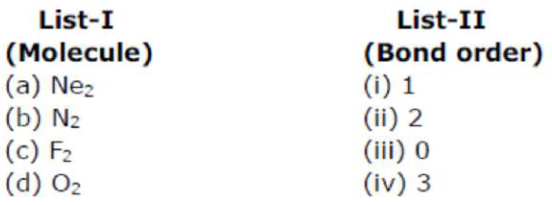# Match List-I with List-II.Question:

Match List-I with List-II.Choose the correct answer from the options given below:

1. $(\mathrm{a})-(\mathrm{iii}),(\mathrm{b})-(\mathrm{iv}),(\mathrm{c})-(\mathrm{i}),(\mathrm{d})-(\mathrm{iii})$

2. (a) $-($ i $),($ b $)-($ ii $),($ c $)-($ iii $),($ d $)-($ iv $)$

3. $(\mathrm{a})-(\mathrm{ii}),(\mathrm{b})-(\mathrm{i}),(\mathrm{c})-(\mathrm{i} v),(\mathrm{d})-(\mathrm{iii})$

4. $(\mathrm{a})-(\mathrm{i} v),(\mathrm{b})-(\mathrm{iii}),(\mathrm{c})-(\mathrm{ii}),(\mathrm{d})-(\mathrm{i})$

Correct Option:

Solution:

$\mathrm{Ne}_{2} \mathrm{O} \quad \mathrm{BO}=0$

$\mathrm{N}_{2} \mathrm{BO}=3$

$\mathrm{F}_{2} \quad \mathrm{BO}=1$

$\mathrm{O}_{2} \quad \mathrm{BO}=2$

As per molecular orbital theory